In a good solvent, when polymer molecules get dissolved, relatively extended conformations with a longer end-to-end distance are more common than solvent–solvent or segment–segment affinities.

A polymer molecule shrinks as the segment–solvent affinity decreases, lowering the end-to-end distance.

Because molecules with a significant end-to-end distance take up more space, they produce a viscous solution.

Thus, the intrinsic viscosity can be used to estimate the state of expansion of a polymer molecule [η].

The intrinsic viscosity is a measure of the molecular expansion of a polymer chain with a size of m3/kg (effective volume per unit mass).

Let us learn what intrinsic viscosity is. And its applications further in this article.

## Introduction

One of the fundamental properties of polymers in dilute solution is intrinsic viscosity [η], which is a measure of a polymer's ability to increase the viscosity of a solvent.

It is widely used in material, engineering, and biology to determine polymers' molecular weight, size, and topological structure.

The Intrinsic Viscosity of polymers has been researched virtually from the inception of the field, and it is a key component of modern molecular theory.

Intrinsic viscosity was formerly the focus of famous physicists like Einstein and Debye and pioneers in polymer physics like Flory, Rouse, Zimm, and Yamakawa, to name a few.

Due to the peculiar behavior of rings, stars, hyperbranched polymers, and dendrimers in comparison to their linear counterparts, and the growing need to characterize hyperbranched polymers and dendrimers, as well as biomacromolecules such as proteins and polysaccharides, the subject has received renewed experimental and theoretical attention in recent years.

Einstein estimated the viscosity of a dilute suspension of impermeable spheres, which was the first molecular theory of viscosity.

However, unlike an impermeable sphere, the solvent can partially flow through a polymer coil; the extent of this partial flow is determined by the monomer density profile, and its first-principles computation is a multibody issue that cannot be addressed using available theories.

The difficulty of explaining the flow of a viscous fluid through a coiled polymer molecule has piqued the interest of theoreticians in numerous fields of chemical physics and biology.

Debye and Brinkman each looked into a molecular model that consisted of a sphere with an evenly distributed system of resisting points.

They got some qualitative responses that corroborated the experimental findings.

Because of the oversimplified treatment for monomer density profile, their methods did not provide a quantitative description of the Intrinsic Viscosity of polymers.

By characterizing the solvent flow through the polymer coil as a porous sphere with a spherically symmetric distribution of permeability dictated by the local density, Wiegel constructed a theory for the intrinsic viscosity of linear and star polymers.

However, such a technique failed to account for long-range, accumulated hydrodynamic effects, which would typically necessitate a nonlocal permeability-density relationship.

Furthermore, the monomers' finite volume was not taken into account.

The volume effects are particularly critical for hyperbranched polymers or dendrimers, whose interior density can reach incredibly high levels.

## What is Intrinsic Viscosity?

Intrinsic Viscosity (IV) measures a polymer's molecular weight and reflects the material's crystallinity, melting point, and tensile strength.

"A measure of a solute's contribution to the viscosity of a solution," or, more precisely, an increase in viscosity caused by the addition of an infinitesimal amount of solute to a solvent is used to define intrinsic viscosity.

In the disciplines of polymer and protein research, intrinsic viscosity is a useful tool.

Intrinsic viscosity provides for a more accurate estimation of molecule weight and size than the standard method of determining these properties, dynamic light scattering.

Polymers have different qualities depending on the type of monomer, the size, and the molecular structure of the polymer molecule.

There are numerous polymer characterization techniques available. One of them is "dilute solution viscometry," which can be used to determine factors like intrinsic viscosity.

You must first measure the viscosity of your solution at various concentrations before applying the Huggins and Kraemer equation to estimate the Intrinsic Viscosity.

The intrinsic viscosity of a solute's molecular interaction with a solvent is a reliable and sensitive indicator that can be used to detect the molecular characteristics of a sample, such as:

• Molecular size and weight

• Quality control tool for polymers

• Polymerization

• Examines polymer architecture (branching)

• Determine viscosity and average molecular weight of polymers

• Interaction of molecules

• Branching structure

• Stability of molecules: denaturation, aggregation, or conformational changes of protein molecules

• Protein structure and melting temperatures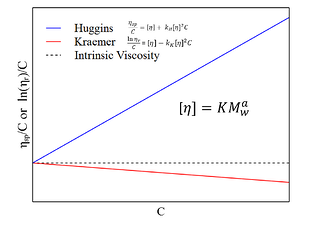The hypothesized intrinsic viscosity of a solution at infinite dilution is called intrinsic viscosity. It's possible to write it as: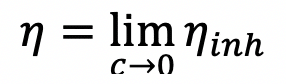Where η denotes the solute's Intrinsic Viscosity, c represents the solute's concentration, and ηinh represents the solution's inherent viscosity.

The Mark-Houwink equation can be used to determine the molecular weight of a polymer using intrinsic viscosity.

Numerous solute solutions at various concentrations are created to determine the intrinsic viscosity.

Each solution's Intrinsic Viscosity is then measured and shown as a function of concentration.

The inherent viscosity is the y-intercept of this graph. Intrinsic viscosity is measured in deciliters per gram (dL/g), or inverse concentration, and can be seen as a measure of a polymer's molecular density under specific conditions (solvent composition and temperature).

## Intrinsic Viscosity (IV) Measurement Methods

### Dilute solution viscometry

Dilute solution viscometry in polymer chemistry is a well-known analytical technique for characterizing polymers in solution.

The concept of this method is that dissolving a polymer in a solvent causes the resulting polymer solution to become viscous.

The temperature, the kind of polymer and solvent, the molar mass of the polymer, and the concentration of the polymer all influence viscosity.

Generally speaking, the larger the molar mass of a polymer, the higher the viscosity of the polymer solution.

The viscosity of the pure solvent when related to the viscosity of the polymer solution (polymer dissolved in solvent), resulting in a relative viscosity value.

As demonstrated in Figure 2, many polymer parameters can be determined using this basic parameter.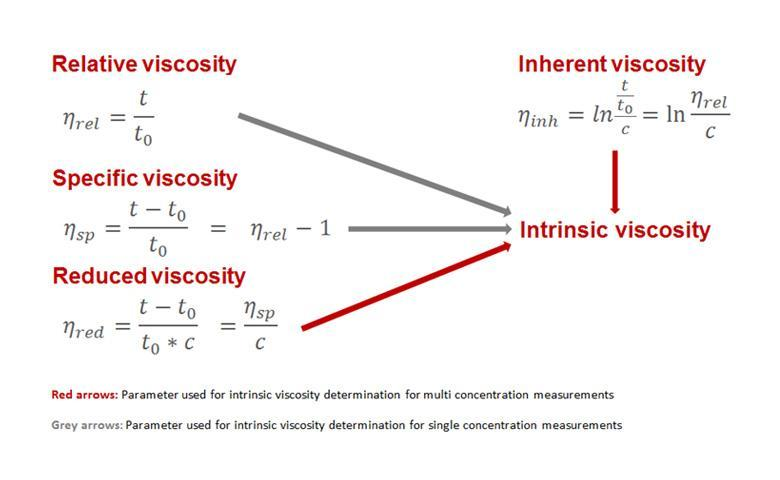Figure 2: Calculating the intrinsic viscosity using the relative viscosity

The polymer's molecular structure, as well as the interactions of the molecules in the solution, have a significant impact on the flow behavior of the polymer solution.

Viscosity measurements are done with very dilute solutions because molecular interactions decrease with decreasing concentrations.

Only the state of "a perfect dilute solution" may produce a solution free of all interactions between polymer molecules.

The concentration approaches zero in this "ideal dilute solution," implying that the polymer molecules are isolated from one another and only interact with the solvent molecules.

This state, however, can never be reached in reality. Therefore tiny polymer interactions must be considered.

The concentration value is required for both the decreased and inherent viscosity calculations.

Thus, intrinsic viscosity is a significant parameter because it represents an extrapolation to a theoretical zero concentration.

The inherent viscosity and other polymer properties are determined using a variety of glass capillary viscometers, particularly Ubbelohde-type glass capillary viscometers.

Alternatives include falling-ball and rolling-ball viscometers. Compared to Ubbelohde viscometers, the rolling-ball viscometer has several advantages.

It uses less energy and less solvent and sample and has a smaller footprint. In addition, the system is closed and may be automated, making user handling much safer, more efficient, and convenient.

The Intrinsic Viscosity can be determined in two methods, depending on the equipment employed, as explained in the following paragraphs.

### Single-concentration Measurements

Multi-concentration measurements take a long time since sample preparation for several polymer solutions must be completed first, followed by viscosity determination for the various polymer solutions.

As a result, the intrinsic viscosity can be derived from a single polymer solution, especially when the intrinsic viscosity is estimated.

Most of the equations utilized are simplified versions of multi-concentration equations derived from relative and specific viscosity (Figure 3).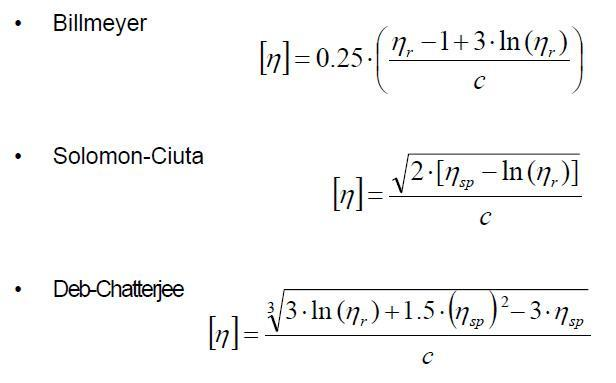Figure 3: When measuring one single polymer solution, intrinsic viscosity calculations equations.  Billmeyer,  Solomon-Ciuta,  Deb-Chatterjee

### Multi-concentration Measurements

Polymer solutions of various concentrations are created for multi-concentration measurements.

These solutions establish the particular, reduced, or intrinsic viscosity based on the regression results.

The calculated viscosity is then displayed versus the concentration of the polymer solutions under investigation.

The intersection determines the Intrinsic Viscosity with the y-axis.

The relative viscosities of the examined polymer solutions should be between 1.2 and 2.5 to ensure an accurate analysis.

Different regression models might be utilized depending on the type of polymer being studied. Figure 4 shows some examples of regressions.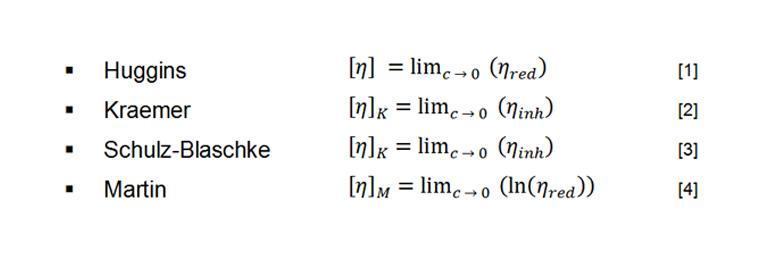Figure 4: Equations for intrinsic viscosity calculations when measuring several polymer solutions of different concentrations. Huggins , Kraemer , Schulz-Blaschke , Martin 

It must first be determined which equation applies to a certain polymer-solvent combination before it can be used.

Figure 5 illustrates an extrapolation of decreasing viscosity to zero concentration using the Huggins equation (Figure 4, ).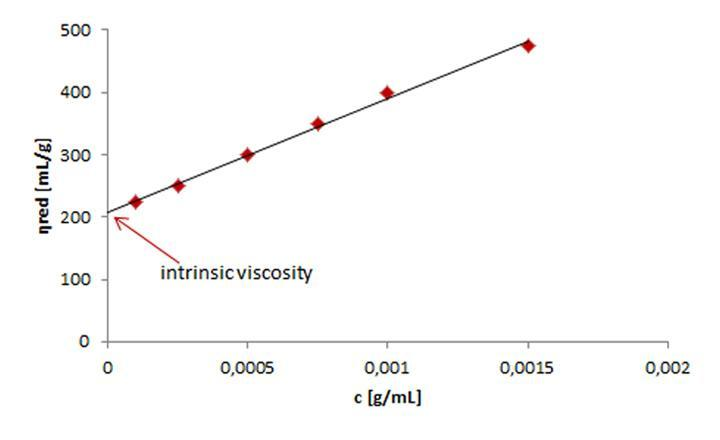Figure 5: Intrinsic viscosity calculation using extrapolation of the reduced viscosity of six polymer solutions to zero concentration.

### Intrinsic Viscosity Calculation

The polymer solution’s intrinsic viscosity is the most important characteristic in describing its viscous behavior.

This is because intrinsic viscosity determines a polymer's genuine viscosity-enhancing characteristics regardless of its concentration in solution.

It's a term that's used in a variety of sectors to define a product's storage stability and quality.

The plastics sector is one example of where intrinsic viscosity is used. In the pharmaceutical sector, however, inherent viscosity is an important testing criterion.

Viscosity measurements for estimating Intrinsic Viscosity should always be performed at a shear rate close to zero.

The viscosity becomes shear-dependent at high shear rates as the polymer solutions begin to exhibit non-Newtonian behavior, which is not the case at low shear rates (zero-shear viscosity).

At various shear rates, polymer solutions can be measured to estimate the intrinsic viscosity at a shear rate approaching zero.

The resulting viscosity values are then extrapolated.

The intersection determines the zero-shear intrinsic viscosity with the y-axis (Figure 6).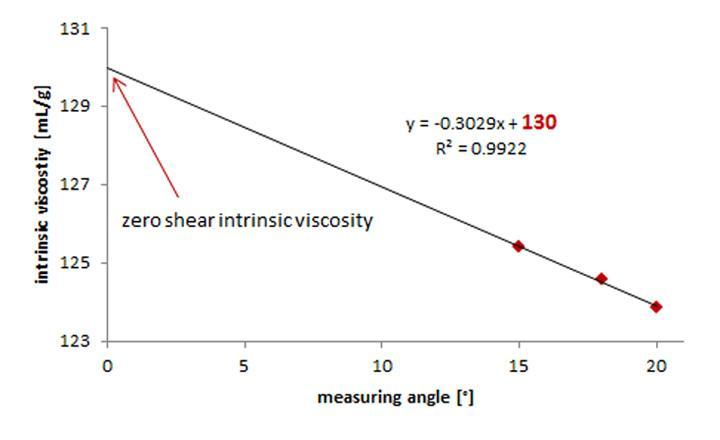Figure 6: Intrinsic viscosity at a theoretical zero shear rate Determination

### Intrinsic Viscosity and Molar Mass

The Mark-Houwink equation can be used to connect the intrinsic viscosity of a polymer in a given solvent with its molar mass.

The intrinsic viscosity formula as per the Mark-Houwink equation is -

[η]=KMa[η] =KMa

[η][η] = intrinsic viscosity
K, a = Mark-Houwink constants

K and a are temperature constants for a particular polymer-solvent combination. If they aren't known from the literature, they must be calculated empirically by charting the logarithm of intrinsic viscosity as a function of logarithmic molar mass (Figure 7).

The constant log K is obtained by intersecting the y-axis of a linear fit of data, and the slope of the curve yields the constant “a” (△x/△y).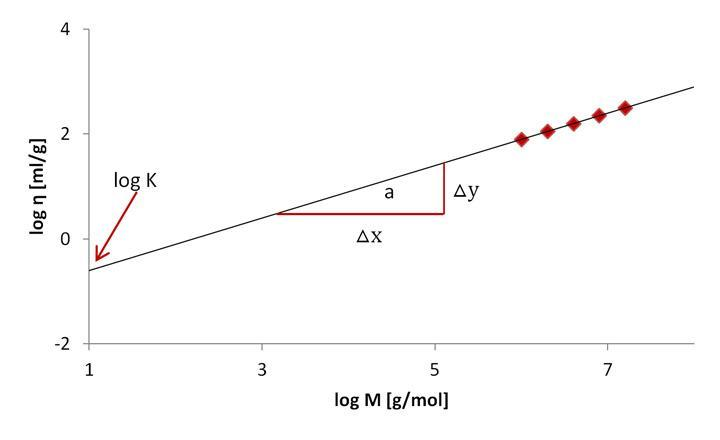Figure 7: K and “a” Determination from a linear plot of the intrinsic viscosity against the molar mass

Examples of K and “a” for some polymer-solvent combinations are given in Table 1.

 Polymer/Solvent Temperature K x10^5 [dL/g] a Polypropylene/Cyclohexane 25 °C 3.97 0.820 Hyaluronic Acid/0.2 M NaCl 25 °C 22.8 0.816 Cellulose Acetate/Water 25 °C 20.9 0.600 Natural rubber/THF 25 °C 10.9 0.790 Polystyrene/Toluene 20 °C 11.2 0.722

Table 1: selected polymer-solvent K and “a” combinations

## Applications

The intrinsic viscosity of spheroids, particularly prolate spheroids, is highly dependent on their axial ratio.

The intrinsic viscosity, for example, can be used to estimate the number of subunits in a protein fiber made up of a helical array of proteins like tubulin.

Intrinsic viscosity can also be used to test a quaternary structure in general.

The Mark–Houwink equation is used in polymer chemistry to relate Intrinsic Viscosity to molar mass.

A Ubbelohde viscometer is a practical method for determining intrinsic viscosity.

### Industries Where Intrinsic Viscosity is Important:

• Food Polymers (Hydrodynamic Volume of Biopolymers)

• Polymers/Coatings (R&D, QC)

• Protein Formulation

• Research Institutions

## Conclusion

One of the most commonly determined characteristics used to characterize polymers is inherent viscosity.

It has a wide range of applications, including quality control in plastic manufacturing and recycling and quality control of substances used in the pharmaceutical business.

Dilute solution viscometry is a popular analytical approach in polymer chemistry because it is inexpensive, simple to perform, and exact.

As a result, it may be found in many laboratories worldwide, and various ISO and ASTM standards for evaluating a wide range of polymers are available.

We hope that you now have a good understanding of Intrinsic Viscosity and its applications. If you still want to know about it or have any queries, feel free to contact Svan